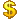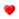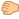ClixBlue.com Forum
21 replies to this topicdragon
 Standard Posts: 25
Le 22/11/2017

RR = 24
clics = 44
gains = 0.154 \$

RD = 2
Clics = 4
Gains = 0.002 \$

Moi = 26 clics
Gains = 0.028 \$

TTC = 0.184 \$
« Last Edit: 2017-11-24 at 20:12 by dragon »dragon
 Standard Posts: 25
Le 23/11/2017

RR = 24
clics = 46
gains = 0.161 \$

RD = 2
Clics = 4
Gains = 0.002 \$

Moi = 26 clics
Gains = 0.028 \$

TTC = 0.191 \$dragon
 Standard Posts: 25
Le 24/11/2017

RR = 27 +3
clics = 48
gains = 0.168 \$

RD = 2
Clics = 4
Gains = 0.002 \$

Moi = 27 clics
Gains = 0.0262 \$

TTC = 0.1962 \$dragon
 Standard Posts: 25
Le 25/11/2017

RR = 27
clics = 54
gains = 0.189 \$

RD = 2
Clics = 0
Gains = 0.000 \$

Moi = 29 clics
Gains = 0.0225 \$

TTC = 0.2115 \$dragon
 Standard Posts: 25
Le 26/11/2017

RR = 27
clics = 53
gains = 0.1855 \$

RD = 2
Clics = 4
Gains = 0.002 \$

Moi = 35 clics
Gains = 0.0376 \$

TTC = 0.2251 \$dragon
 Standard Posts: 25
Le 27/11/2017

RR = 27
clics = 52
gains = 0.260 \$

RD = 2
Clics = 0
Gains = 0.000 \$

Moi = 35 clics
Gains = 0.0592 \$

TTC = 0.3192 \$dragon
 Standard Posts: 25
Le 28/11/2017

RR = 27
clics = 28
gains = 0.14 \$

RD = 2
Clics = 0
Gains = 0.000 \$

Moi = 35 clics
Gains = 0.0592 \$

TTC = 0.1992 \$dragon
 Standard Posts: 25
Le 29/11/2017

RR = 27
clics = 29
gains = 0.145RD = 2
Clics = 4
Gains = 0.0025Moi = 37 clics
Gains = 0.0592TTC = 0.2142dragon
 Standard Posts: 25
Le 30/11/2017

RR = 27
clics = 28
gains = 0.140RD = 2
Clics = 8
Gains = 0.020Moi = 37 clics
Gains = 0.0592TTC = 0.2192dragon
 Standard Posts: 25
Le 01/12/2017

RR = 27
clics = 28
gains = 0.140RD = 2
Clics = 0
Gains = 0.000Moi = 36 clics
Gains = 0.0592TTC = 0.1992